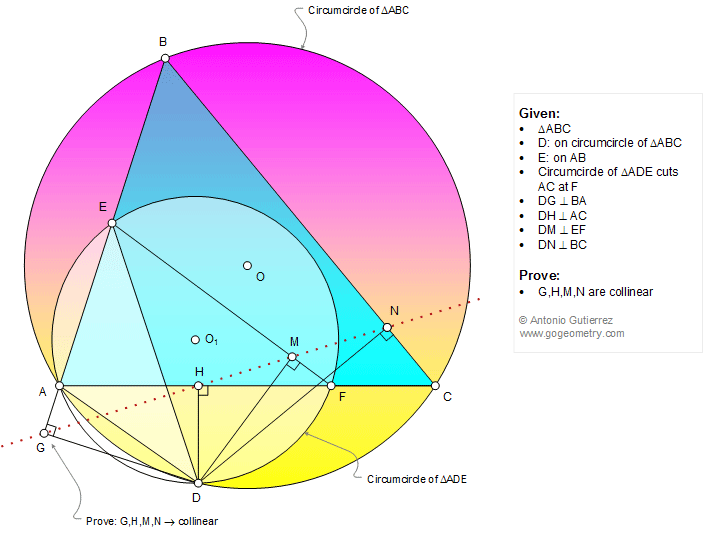# Geometry Problem 1167: Triangle, Circle, Circumcircle, Perpendicular, 90 Degrees, Collinear Points. Level: High School, Honors Geometry, College, Mathematics Education

< PREVIOUS PROBLEM  |  NEXT PROBLEM >

D is a point on the circumcircle of a triangle ABC and E is a point on AB (see figure below). The circumcircle of triangle ADE cuts AC at F. If DG, DH, DM, and DN are perpendicular to BA, AC, EF, and BC, respectively, prove that points G, H, M, and N are collinear.